# TensorFlow从1到2（十四）#### 三种开发模式

• 使用底层逻辑
• 使用Keras高层接口
TensorFlow 1.x的开发中，Keras就作为第三方库存在。2.0中，更是已经成为标准配置。我们前面大多的例子都是基于Keras或者自定义Keras模型配合底层训练循环完成。从网上的一些开源项目来看，这已经是应用最广泛的方式。
• 今天要介绍的评估器tf.estimator
评估器是TensorFlow官方推荐的内置高级API，层次上看跟Keras实际处于同样位置，只是似乎大家都视而不见了，以至于现在从用户的实际情况看用的人要远远少于Keras。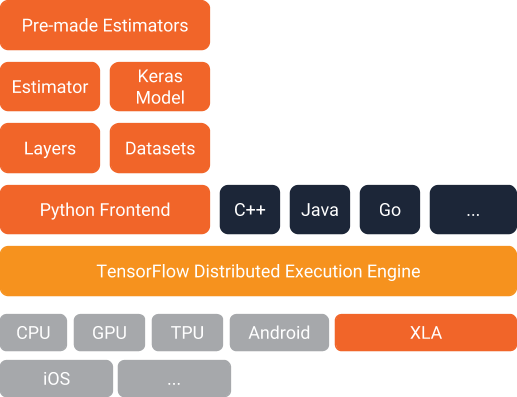通常认为评估器因为内置的紧密结合，运行速度要高于Keras。Keras一直是一个通用的高层框架，除了支持TensorFlow作为后端，还同时支持Theano和CNTK。高度的抽象肯定会影响Keras的速度，不过本人并未实际对比测试。我觉的，对于大量数据导致的长时间训练来说，这点效率上的差异不应当成为大问题，否则Python这种解释型的语言就不会成为优选的机器学习基础平台了。

• 载入数据
• 数据清洗和数据预处理
• 编写数据流水线输入函数
• 定义评估器模型
• 训练
• 评估

#### 使用评估器的实例

sex 乘客性别
age 乘客年龄
n_siblings_spouses 随行兄弟或者配偶数量
parch 随行父母或者子女数量
fare 船费金额
class 船舱等级
deck 甲板编号
embark_town 登船地点
alone 是否为独自旅行

#### 基本数据分析

``````# 引入扩展库
from __future__ import absolute_import, division, print_function, unicode_literals

import numpy as np
import pandas as pd
import matplotlib.pyplot as plt
import tensorflow as tf

# 载入数据
# 分离标注字段
y_train = dftrain.pop('survived')
y_eval = dfeval.pop('survived')

``````

``````\$ python3
Python 3.7.3 (default, Mar 27 2019, 09:23:39)
[Clang 10.0.0 (clang-1000.11.45.5)] on darwin
>>> # 引入扩展库
... from __future__ import absolute_import, division, print_function, unicode_literals
>>>
>>> import numpy as np
>>> import pandas as pd
>>> import matplotlib.pyplot as plt
>>> import tensorflow as tf
>>>
>>> # 载入数据
>>> # 分离标注字段
... y_train = dftrain.pop('survived')
>>> y_eval = dfeval.pop('survived')
>>>
sex   age  n_siblings_spouses  parch     fare  class     deck  embark_town alone
0    male  22.0                   1      0   7.2500  Third  unknown  Southampton     n
1  female  38.0                   1      0  71.2833  First        C    Cherbourg     n
2  female  26.0                   0      0   7.9250  Third  unknown  Southampton     y
3  female  35.0                   1      0  53.1000  First        C  Southampton     n
4    male  28.0                   0      0   8.4583  Third  unknown   Queenstown     y
>>>
``````

``````dftrain.age.hist(bins=20)
plt.show()
``````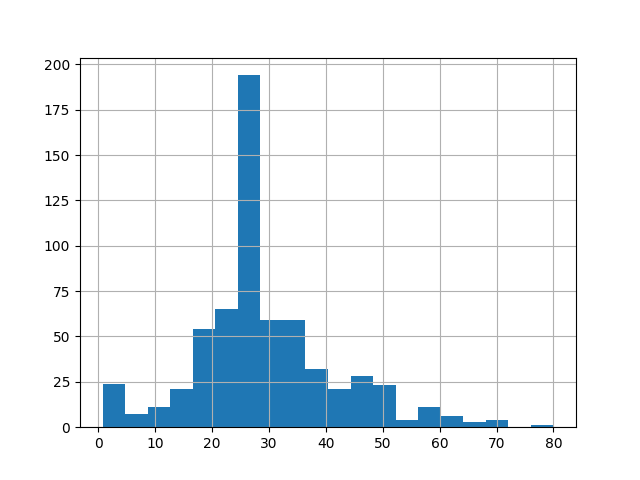直方图中显示，乘客年龄主要分布在20岁至30岁之间。

``````dftrain.sex.value_counts().plot(kind='barh')
plt.show()
``````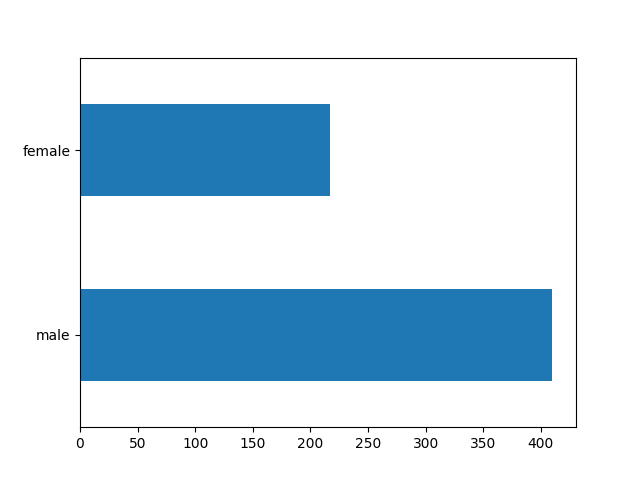男性乘客的数量，几乎是女性乘客的两倍。

``````dftrain['class'].value_counts().plot(kind='barh')
plt.show()
``````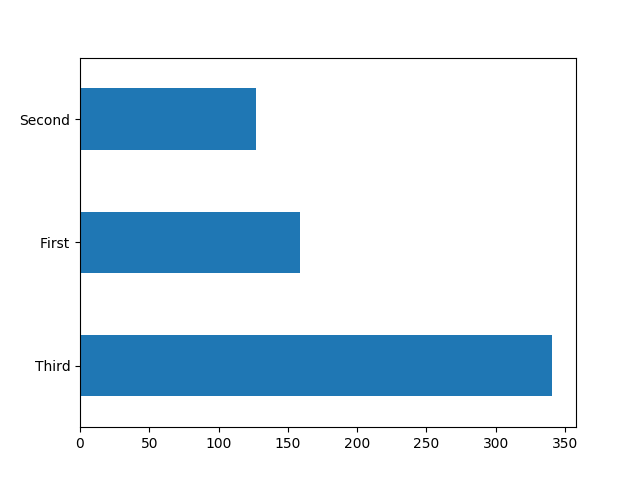图中显示，大多数乘客还是在三等舱。

``````dftrain['embark_town'].value_counts().plot(kind='barh')
plt.show()
``````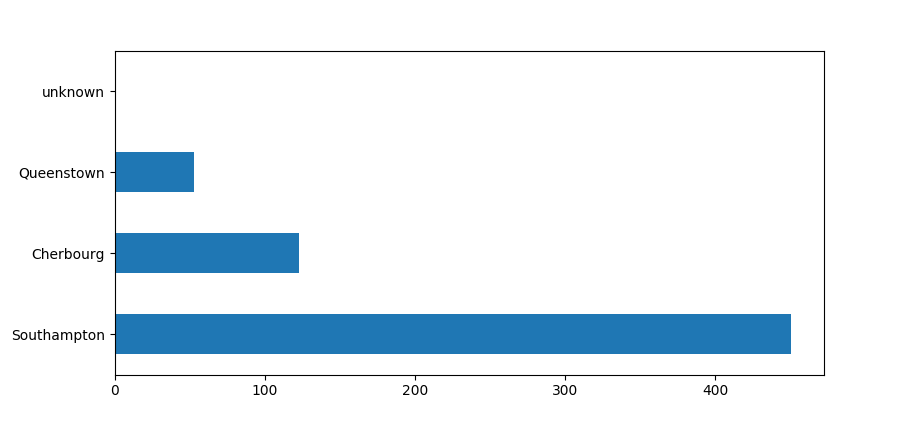大多数乘客来自南安普顿。

``````pd.concat([dftrain, y_train], axis=1).groupby('sex').survived.mean().plot(kind='barh').set_xlabel('% survive')
plt.show()
``````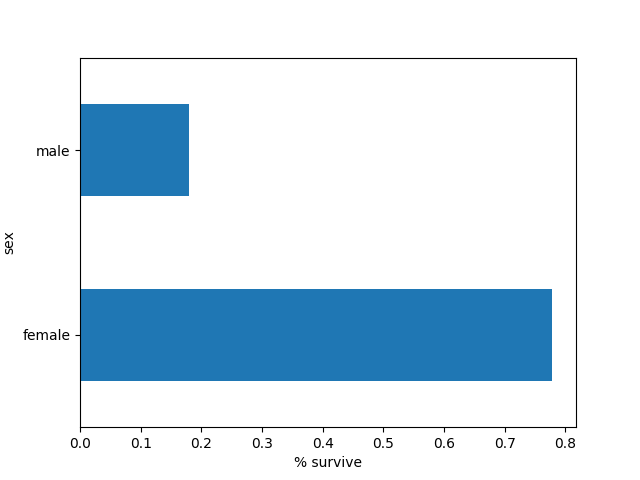女性的存活率几乎超过男性的5倍。

``````def calc_age_section(n, lim):
return'[%.f,%.f)' % (lim*(n//lim), lim*(n//lim)+lim)  # map function

addone = pd.Series([calc_age_section(s, 10) for s in dftrain.age])
pd.concat([dftrain, y_train], axis=1).groupby('ages').survived.mean().plot(kind='barh').set_xlabel('% survive');
plt.show()

``````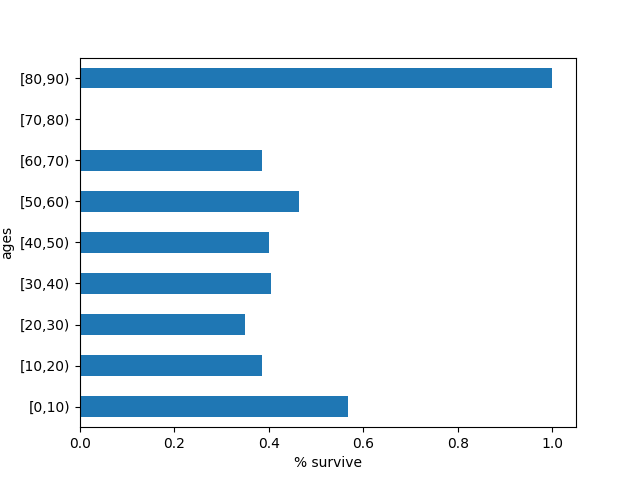10岁以下儿童和80岁以上的老人得到了最多的生存机会。

#### 数据的预处理

``````# 定义所需的数据列，分为分类型属性和数值型属性分别定义
CATEGORICAL_COLUMNS = ['sex', 'n_siblings_spouses', 'parch', 'class', 'deck',
'embark_town', 'alone']
NUMERIC_COLUMNS = ['age', 'fare']

# 辅助函数，把给定数据列做one-hot编码
def one_hot_cat_column(feature_name, vocab):
return tf.feature_column.indicator_column(
tf.feature_column.categorical_column_with_vocabulary_list(feature_name,
vocab))

# 最终使用的数据列，先置空
feature_columns = []
for feature_name in CATEGORICAL_COLUMNS:
# 分类的属性都要做one-hot编码，然后加入数据列
vocabulary = dftrain[feature_name].unique()
feature_columns.append(one_hot_cat_column(feature_name, vocabulary))

for feature_name in NUMERIC_COLUMNS:
# 数值类的属性直接入列
feature_columns.append(tf.feature_column.numeric_column(feature_name,
dtype=tf.float32))
``````

#### 数据输入函数

``````# 这是一个很少量数据的样本，直接把整个数据集当做一批
NUM_EXAMPLES = len(y_train)
# 输入函数的构造函数
def make_input_fn(X, y, n_epochs=None, shuffle=True):
def input_fn():
dataset = tf.data.Dataset.from_tensor_slices((dict(X), y))
# 乱序
if shuffle:
dataset = dataset.shuffle(NUM_EXAMPLES)
# 训练时让数据重复尽量多的次数
dataset = dataset.repeat(n_epochs)
dataset = dataset.batch(NUM_EXAMPLES)
return dataset
return input_fn

# 训练、评估所使用的数据输入函数，区别只是数据是否乱序以及迭代多少次
train_input_fn = make_input_fn(dftrain, y_train)
eval_input_fn = make_input_fn(dfeval, y_eval, shuffle=False, n_epochs=1)

``````

#### 模型和源码

``````# 使用线性分类器作为模型
linear_est = tf.estimator.LinearClassifier(feature_columns)

# 训练
linear_est.train(train_input_fn, max_steps=100)

# 评估
result = linear_est.evaluate(eval_input_fn)
``````

``````#!/usr/bin/env python3

# 引入扩展库
from __future__ import absolute_import, division, print_function, unicode_literals

import numpy as np
import pandas as pd
import matplotlib.pyplot as plt
import tensorflow as tf

# 载入数据
# 分离标注字段
y_train = dftrain.pop('survived')
y_eval = dfeval.pop('survived')

################################################################
# 定义所需的数据列，分为分类型属性和数值型属性分别定义
CATEGORICAL_COLUMNS = ['sex', 'n_siblings_spouses', 'parch', 'class', 'deck',
'embark_town', 'alone']
NUMERIC_COLUMNS = ['age', 'fare']

# 辅助函数，把给定数据列做one-hot编码
def one_hot_cat_column(feature_name, vocab):
return tf.feature_column.indicator_column(
tf.feature_column.categorical_column_with_vocabulary_list(feature_name,
vocab))

# 最终使用的数据列，先置空
feature_columns = []
for feature_name in CATEGORICAL_COLUMNS:
# 分类的属性都要做one-hot编码，然后加入数据列
vocabulary = dftrain[feature_name].unique()
feature_columns.append(one_hot_cat_column(feature_name, vocabulary))

for feature_name in NUMERIC_COLUMNS:
# 数值类的属性直接入列
feature_columns.append(tf.feature_column.numeric_column(feature_name,
dtype=tf.float32))

################################################################
# 这是一个很少量数据的样本，直接把整个数据集当做一批
NUM_EXAMPLES = len(y_train)
# 输入函数的构造函数
def make_input_fn(X, y, n_epochs=None, shuffle=True):
def input_fn():
dataset = tf.data.Dataset.from_tensor_slices((dict(X), y))
# 乱序
if shuffle:
dataset = dataset.shuffle(NUM_EXAMPLES)
# 训练时让数据重复尽量多的次数
dataset = dataset.repeat(n_epochs)
dataset = dataset.batch(NUM_EXAMPLES)
return dataset
return input_fn

# 训练、评估所使用的数据输入函数，区别只是数据是否乱序以及迭代多少次
train_input_fn = make_input_fn(dftrain, y_train)
eval_input_fn = make_input_fn(dfeval, y_eval, shuffle=False, n_epochs=1)

# 使用线性分类器作为模型
linear_est = tf.estimator.LinearClassifier(feature_columns)

# 训练
linear_est.train(train_input_fn, max_steps=100)

# 评估
result = linear_est.evaluate(eval_input_fn)
print("----------------------------------")
print(pd.Series(result))
``````

``````----------------------------------
accuracy                  0.765152
accuracy_baseline         0.625000
auc                       0.832844
auc_precision_recall      0.789631
average_loss              0.478908
label/mean                0.375000
loss                      0.478908
precision                 0.703297
prediction/mean           0.350790
recall                    0.646465
global_step             100.000000
``````

``````# 以下代码放在程序最后，因为这个数据集非常小，速度很快，所以做两次学习也并不感觉慢
n_batches = 1
est = tf.estimator.BoostedTreesClassifier(feature_columns,
n_batches_per_layer=n_batches)

# 训练
est.train(train_input_fn, max_steps=100)

# 评估
result = est.evaluate(eval_input_fn)
print("----------------------------------")
print(pd.Series(result))

``````

``````----------------------------------
accuracy                  0.825758
accuracy_baseline         0.625000
auc                       0.872360
auc_precision_recall      0.857325
average_loss              0.411853
label/mean                0.375000
loss                      0.411853
precision                 0.784946
prediction/mean           0.382282
recall                    0.737374
global_step             100.000000
``````

#### 性能评价

``````# 绘制预测概率直方图
pred_dicts1 = list(linear_est.predict(eval_input_fn))
pred_dicts2 = list(bt_est.predict(eval_input_fn))
probs1 = pd.Series([pred['probabilities'] for pred in pred_dicts1])
probs2 = pd.Series([pred['probabilities'] for pred in pred_dicts2])

plt.figure(figsize=(14, 5))
plt.subplot(1, 2, 1)
probs1.plot(kind='hist', bins=20, title='linear-est predicted probabilities');
plt.subplot(1, 2, 2)
probs2.plot(kind='hist', bins=20, title='bt-est predicted probabilities');
plt.show()
``````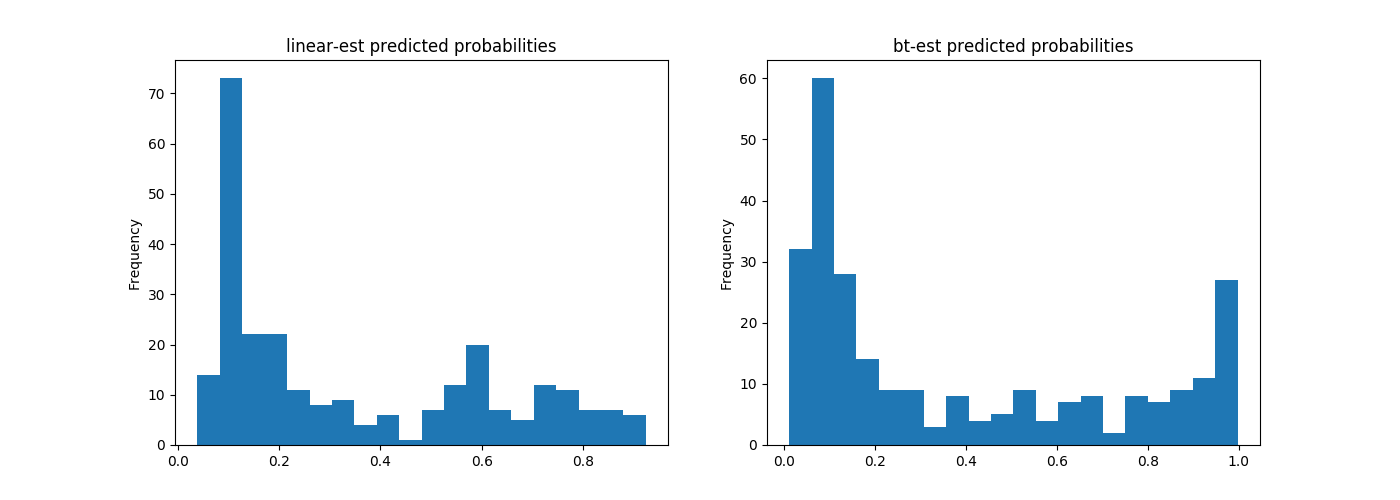大量集中在图形左侧的数据簇，显示了乘客九死一生的悲惨命运。

1. 真阳性（TP）：判断为1，实际上也为1。
2. 伪阳性（FP）：判断为1，实际上为0。
3. 真阴性（TN）：判断为0，实际上也为0。
4. 伪阴性（FN）：判断为0，实际上为1。

ROC图中，左上角是真阳性的极点，曲线越接近左上角，意味着分类器性能越好。所以左上角是分类器追求的方向。

``````# 绘制ROC(Receiver Operating Characteristic)曲线
from sklearn.metrics import roc_curve

def plot_roc(probs, title):
fpr, tpr, _ = roc_curve(y_eval, probs)
plt.plot(fpr, tpr)
plt.title(title)
plt.xlabel('false positive rate')
plt.ylabel('true positive rate')
plt.xlim(0,)
plt.ylim(0,)
plt.figure(figsize=(14, 5))
plt.subplot(1, 2, 1)
plot_roc(probs1, "linear-est ROC")
plt.subplot(1, 2, 2)
plot_roc(probs2, "bt-est ROC")
plt.show()
``````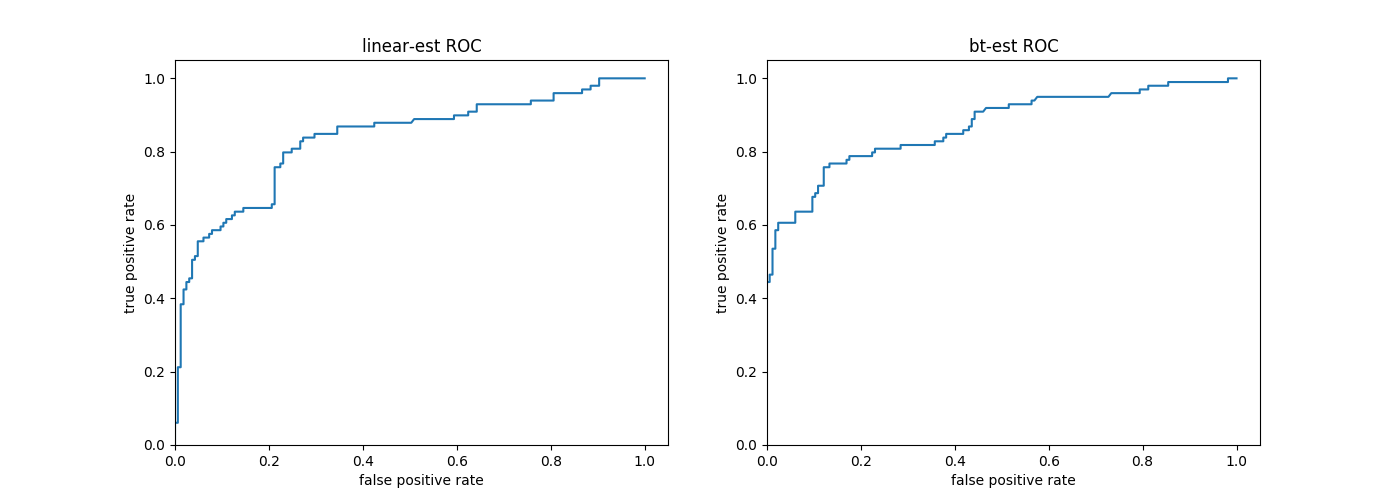从ROC曲线看，在本例中使用提升树模型的优势更为明显。

（待续…）